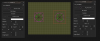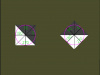# SOLVEDHow to Rotate a 2d Vertex Custom Primitive About A Point

#### Game_Re_creation_Project

##### Member
I can't quite find the answer I'm looking for in the forums.

I am looking for a method to rotate a set of 2d vertices about the object's current origin. Is there a way to do this without using a matrix? (Or even a surface...)

Create:
GML:
``````vertex_format_begin();
v_format = vertex_format_end();
v_buff = vertex_create_buffer();

var currBuffer, currTexture;

currBuffer = v_buff;
currTexture = texture_to_use;
vertex_begin(currBuffer,v_format);
vertex_position(currBuffer,scrollbar_x1+(scrollbar_width*0),scrollbar_y1);
vertex_color(currBuffer,c_dkgray,1.0);
vertex_position(currBuffer,scrollbar_x1+(scrollbar_width*0),scrollbar_y2);
vertex_color(currBuffer,c_dkgray,1.0);
vertex_position(currBuffer,scrollbar_x2+(scrollbar_width*0),scrollbar_y2);
vertex_color(currBuffer,c_dkgray,1.0);

vertex_position(currBuffer,scrollbar_x1+(scrollbar_width*0),scrollbar_y1);
vertex_color(currBuffer,c_dkgray,1.0);
vertex_position(currBuffer,scrollbar_x2+(scrollbar_width*0),scrollbar_y2);
vertex_color(currBuffer,c_dkgray,1.0);
vertex_position(currBuffer,scrollbar_x2+(scrollbar_width*0),scrollbar_y1);
vertex_color(currBuffer,c_dkgray,1.0);

v_buff = currBuffer;
vertex_end(v_buff);
vertex_freeze(v_buff);``````
Draw:
Code:
``vertex_submit(v_buff, pr_trianglelist, -1);``
I assume I would have to run a begin_ and end_step event and check my current rotation to a previous rotation. If there is a change, I would have to unfreeze the vertices and redraw and then re-freeze. That would be the easy part. I'm just wondering if there is some fancy built-in function that would already do this, like vertex_submit_ext(v_buff, pr_trianglelist, -1, current_rotation) or something to that effect.

Would using a matrix cause slowdowns if I only need the vertices in 2d space? This is supposed to be a drawn scrollbar that the user can click on and perform different operations depending on where it is clicked. I figured it would be better to "draw" the scrollbar rather than use sprites as I want this to work with multiple resolutions. My goal is to create a series of user controls similar to what you would find in most handles for windows device controls (HWND) objects.

Last edited:

#### rytan451

##### Member
You could create a rotation matrix in two dimensions (so a 2x2 matrix). Identify the positions of the vertices as vectors from the center of rotation (in this case the object's origin). Multiply these 2-dimensional vectors by the rotation matrix. Add the vector from the global origin to the center of rotation, and you have the position of the vertex.

I've created a bit of code below to make this much easier. (If you so wish, you could change the `cos` and `sin` to `dcos` and `dsin[icode] so you can use degrees instead of radians for the [icode]theta` parameter.

GML:
``````/// @func rotate_vertex_around_x(vx, vx, cx, cy, theta)
/// @desc Rotates the vertex (vx, vy) around (cx, cy) by theta radians, and returns the x position of the resulting vertex.

var c = cos(theta),
s = sin(theta);
return dot(argument0 -  argument2, argument1 - argument3, c, -s) + argument2;

/// @func rotate_vertex_around_y(vx, vx, cx, cy, theta)
/// @desc Rotates the vertex (vx, vy) around (cx, cy) by theta radians, and returns the y position of the resulting vertex.

var c = cos(theta),
s = sin(theta);
return dot(argument0 -  argument2, argument1 - argument3, s, c) + argument3;``````

#### Game_Re_creation_Project

##### Member
You could create a rotation matrix in two dimensions (so a 2x2 matrix). Identify the positions of the vertices as vectors from the center of rotation (in this case the object's origin). Multiply these 2-dimensional vectors by the rotation matrix. Add the vector from the global origin to the center of rotation, and you have the position of the vertex.

I've created a bit of code below to make this much easier. (If you so wish, you could change the `cos` and `sin` to `dcos` and `dsin[icode] so you can use degrees instead of radians for the [icode]theta` parameter.

GML:
``````/// @func rotate_vertex_around_x(vx, vx, cx, cy, theta)
/// @desc Rotates the vertex (vx, vy) around (cx, cy) by theta radians, and returns the x position of the resulting vertex.

var c = cos(theta),
s = sin(theta);
return dot(argument0 -  argument2, argument1 - argument3, c, -s) + argument2;

/// @func rotate_vertex_around_y(vx, vx, cx, cy, theta)
/// @desc Rotates the vertex (vx, vy) around (cx, cy) by theta radians, and returns the y position of the resulting vertex.

var c = cos(theta),
s = sin(theta);
return dot(argument0 -  argument2, argument1 - argument3, s, c) + argument3;``````

The "dot" function doesn't exist in GMS2. It has the dot_product(x1,y1,x2,y2) function.

I tried using a matrix and the results were not as expected. I will have to figure out a way to make something similar to your suggestion work.

#### Game_Re_creation_Project

##### Member
You could create a rotation matrix in two dimensions (so a 2x2 matrix). Identify the positions of the vertices as vectors from the center of rotation (in this case the object's origin). Multiply these 2-dimensional vectors by the rotation matrix. Add the vector from the global origin to the center of rotation, and you have the position of the vertex.

I've created a bit of code below to make this much easier. (If you so wish, you could change the `cos` and `sin` to `dcos` and `dsin[icode] so you can use degrees instead of radians for the [icode]theta` parameter.

GML:
``````/// @func rotate_vertex_around_x(vx, vx, cx, cy, theta)
/// @desc Rotates the vertex (vx, vy) around (cx, cy) by theta radians, and returns the x position of the resulting vertex.

var c = cos(theta),
s = sin(theta);
return dot(argument0 -  argument2, argument1 - argument3, c, -s) + argument2;

/// @func rotate_vertex_around_y(vx, vx, cx, cy, theta)
/// @desc Rotates the vertex (vx, vy) around (cx, cy) by theta radians, and returns the y position of the resulting vertex.

var c = cos(theta),
s = sin(theta);
return dot(argument0 -  argument2, argument1 - argument3, s, c) + argument3;``````
I tried this and it mimics the built-in point_distance() and point_direction() functions which I had already implemented; I could already get an object to orbit another object. My interest is in getting vertices to rotate while orbiting so the object always "faces" the point about which it is orbiting.

#### Binsk

##### Member
Use a matrix. The only 'slow' part about using a matrix is building it which still shouldn't be much of an issue and would certainly be faster than manually modifying each vertex. Your graphics card ALREADY uses 3D matrix math to calculate your vertex positions in the first place. Using your own custom matrix adds literally no extra work to your GPU.

Use matrix_build to make your matrix. You are 2D so you can set the z-position to 0. You are still technically rotating around the z-axis, however, so that is the rotation you should specify (both x and y rotations can be 0). All the scales can be set to 1.

If you try manually modifying each vertex through GML then this means all the work is being done on the CPU which is extremely wasteful (and more likely to cause slowdown). Your GPU is designed specifically for matrix math so you should let it do that bit while leaving the rest up to your CPU.

Last edited:
•Bart

#### rytan451

##### Member
I agree with the above. But if you want to make vertices rotate to face a point, you can rotate around that point (using the cx and cy coordinates).

Matrices are really fast. As in, extremely, blindingly fast. It's literally a few dozen multiplications and a handful of additions. Doing the rotation on the graphics card by using a matrix is faster than rotating the vertices in GML. Building the matrix is also blindingly fast. If you're worried about performance, use a matrix and worry about something else.

#### Game_Re_creation_Project

##### Member
Use a matrix. The only 'slow' part about using a matrix is building it which still shouldn't be much of an issue and would certainly be faster than manually modifying each vertex. Your graphics card ALREADY uses 3D matrix math to calculate your vertex positions in the first place. Using your own custom matrix adds literally no extra work to your GPU.

Use matrix_build to make your matrix. You are 2D so you can set the z-position to 0. You are still technically rotating around the z-axis, however, so that is the rotation you should specify (both x and y rotations can be 0). All the scales can be set to 1.

If you try manually modifying each vertex through GML then this means all the work is being done on the CPU which is extremely wasteful (and more likely to cause slowdown). Your GPU is designed specifically for matrix math so you should let it do that bit while leaving the rest up to your CPU.
Hmm, it seems matrices "shift" the current "view" relative to their x,y coordinates. My object kept orbiting at a very wide angle. I moved the object relative to the matrix (well, the world view) and it is now centered based on the current matrix even though the current matrix uses room_get_width/2 and room_get_height/2 for its x and y coordinates. I didn't initially realize that was happening!

This works:
Create:
GML:
``````//Must shift left and up by half of dummy sprite size in room editor
//Dummy sprite is used for placing and rotating the object and is not actually drawn; the mesh is instead drawn
drawn_zero_x = -(sprite_width * image_xscale)/2;
drawn_zero_y = -(sprite_height * image_yscale)/2;
drawn_width_x = (sprite_width * image_xscale)/2;
drawn_height_y = (sprite_height * image_yscale)/2;

ang = 0;

vertex_format_begin();
v_format = vertex_format_end();
v_buff = vertex_create_buffer();

vertex_begin(v_buff,v_format);

vertex_position(v_buff,drawn_zero_x,drawn_zero_y);
vertex_color(v_buff,c_white,1.0);
vertex_position(v_buff,drawn_zero_x,drawn_height_y);
vertex_color(v_buff,c_white,1.0);
vertex_position(v_buff,drawn_width_x,drawn_height_y);
vertex_color(v_buff,c_white,1.0);

vertex_position(v_buff,drawn_zero_x,drawn_zero_y);
vertex_color(v_buff,c_dkgray,1.0);
vertex_position(v_buff,drawn_width_x,drawn_height_y);
vertex_color(v_buff,c_dkgray,1.0);
vertex_position(v_buff,drawn_width_x,drawn_zero_y);
vertex_color(v_buff,c_dkgray,1.0);
vertex_end(v_buff);
vertex_freeze(v_buff);``````
Step:
GML:
``````if ang < 359
ang += 1 ;
else
ang = 0;``````
Draw:
GML:
``````//shift matrix to point x,y and submit vertex which is drawn with its center at the "mid-point" relative to the
//object's origin which is at the center of the room
var t_matrix = matrix_build(x,y,0,0,0,ang,1,1,1);
matrix_set(matrix_world,t_matrix);
vertex_submit(v_buff,pr_trianglelist,-1);
matrix_set(matrix_world,matrix_build_identity());``````
The mesh rotates about the origin! (As it should!)

That was a wicked 48 hours! A quick suggestion for anyone else planning to draw a rotated vertex using matrices: the z-axis rotates about the upper-left corner of the x and y coordinates of the matrix. Hence, if your object was placed at the "center" of the room relative to the matrix_world before creating the temporary matrix, the object will rotate about an unexpected trajectory! Also, be sure to reset the matrix to {[1,0,0,0],[0,1,0,0],[0,0,1,0],[0,0,0,1]} using `matrix_set(matrix_world,matrix_build_identity())` when done, otherwise the new matrix will affect all of the subsequent draws.

Result:#### Game_Re_creation_Project

##### Member
Next question: After the matrix is rotated, how can I ensure that the mouse x and y coordinates still return the correct values based on the current view? Is that process done automatically?

#### Game_Re_creation_Project

##### Member
Update:

Use this code to always position the current mesh in the same position as its object.

Create:
GML:
``````//Position for current mesh
//left
drawn_zero_x = -sprite_xoffset;
//top
drawn_zero_y = -sprite_yoffset;
//right
drawn_width_x = sprite_width - sprite_xoffset;
//bottom
drawn_height_y = sprite_height - sprite_yoffset;

//Current mesh
vertex_format_begin();
v_format = vertex_format_end();
v_buff = vertex_create_buffer();

vertex_begin(v_buff,v_format);

//counter-clockwise winding order
vertex_position(v_buff,drawn_zero_x,drawn_zero_y);
vertex_color(v_buff,c_white,1.0);
vertex_position(v_buff,drawn_zero_x,drawn_height_y);
vertex_color(v_buff,c_white,1.0);
vertex_position(v_buff,drawn_width_x,drawn_height_y);
vertex_color(v_buff,c_white,1.0);

vertex_position(v_buff,drawn_zero_x,drawn_zero_y);
vertex_color(v_buff,c_dkgray,1.0);
vertex_position(v_buff,drawn_width_x,drawn_height_y);
vertex_color(v_buff,c_dkgray,1.0);
vertex_position(v_buff,drawn_width_x,drawn_zero_y);
vertex_color(v_buff,c_dkgray,1.0);
vertex_end(v_buff);
vertex_freeze(v_buff);``````
Draw:
GML:
``````//temp matrix with mesh drawn centered to current object sprite's origin while inhereiting the current object's image_angle
var t_matrix = matrix_build((x + lengthdir_x(0, image_angle)),(y + lengthdir_y(0, image_angle)),0,0,0,image_angle,1,1,1);
matrix_set(matrix_world,t_matrix);
vertex_submit(v_buff,pr_trianglelist,-1);
matrix_set(matrix_world,matrix_build_identity());

//draw the current object
draw_self();``````
Room Editor (One object is rotated by 45-degrees):Runtime Result:The mesh always mimics the object regardless of its origin position.
Hope someone else in the future can benefit from this.